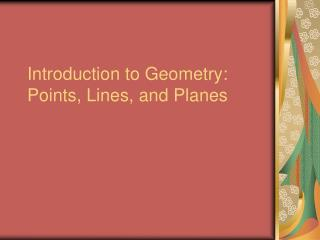DownloadDownload PresentationIntroduction to Geometry: Points, Lines, and Planes

# Introduction to Geometry: Points, Lines, and Planes

Télécharger la présentation## Introduction to Geometry: Points, Lines, and Planes

- - - - - - - - - - - - - - - - - - - - - - - - - - - E N D - - - - - - - - - - - - - - - - - - - - - - - - - - -
##### Presentation Transcript

1. Introduction to Geometry: Points, Lines, and Planes

2. Basic Geometric Figures • Point: Location in space represented by a dot and a capital letter. • Points have no size, only position. • Example:

3. Basic Geometric Figures • Line: Connected series of points that extends in both directions without end (represented by arrows). • Lines can be named by any two points on the line, or by one lowercase letter.

4. Basic Geometric Figures • Plane: Infinite set of points forming a flat surface extending in all directions, represented by a four-sided figure. • Example:

5. Basic Geometric Figures • Segment: Part of a line bounded by two endpoints.

6. Basic Geometric Figures • Ray: Part of a line that starts at a certain (end)point and continues forever in one direction.

7. Naming Geometric Figures • Intersection: The point shared by two crossing figures.

8. Naming Geometric Figures • Parallel: Two lines in the same plane that never intersect. • Represented by symbol “||” (line 1 || line2)

9. Naming Geometric Figures • Skew: Two or more lines that do not lie in the same plane. They are not parallel and do not intersect.

10. Real Life Example E • Find four segments that intersect AC. • Find three segments || to AC. • Find four segments skew to AC. A F B FedEx G C H D